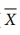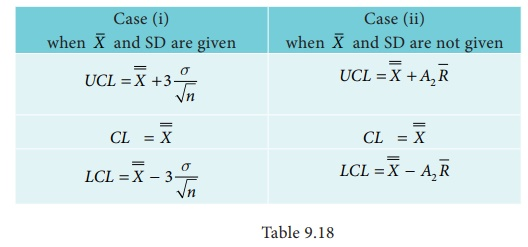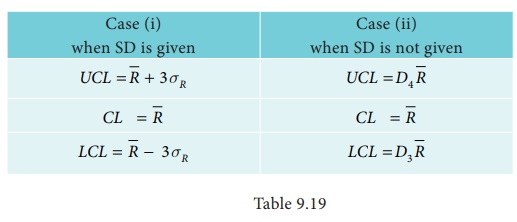Home | | Business Maths 12th Std | Construction of X and R charts

# Construction of X and R charts

Any production process is not perfect enough to produce all the products exactly the same.

Applied Statistics : Statistical Quality Control (SQC)

Construction ofand R charts

Any production process is not perfect enough to produce all the products exactly the same. Some amount of variation is inherent in any production process. This variation is a total of number of characteristics of the production process such as raw materials, machine setting, operators, handling new operations and new machines, etc. Thechart is used to show the quality averages of the samples taken from the given process. The R chart is used to show the variability or dispersion of the samples taken from the given process. The control limits of theand R charts shows the presence or absence of assignable causes in the production process. Bothand R charts are usually required for decision making to accept or reject the process.

The procedure for constructingand R charts are outlined below.

Procedure for(i) Let X1,X2, X3, etc. be the samples selected, each containing ‘n’ observations (usually n = 4, 5 or 6)

(ii) Calculate mean for each samples12 ,3 .... by usingtotal of ‘n’ values included in the  sample Xi .

(iii) Find the mean () of the sample means.total of all the sample means.

## Procedure for R -Charts.

Calculate  R = xmax xmin

Let R1, R2, R3… be the ranges of the ‘n’ samples. The average range is given byThe calculation of control limits forchart in two different cases isThe calculation of control limits for R chart in two different cases areThe values of A2, D3 and D4 are given in the table.

Tags : Statistical Quality Control (SQC) | Applied Statistics , 12th Business Maths and Statistics : Chapter 9 : Applied Statistics
Study Material, Lecturing Notes, Assignment, Reference, Wiki description explanation, brief detail
12th Business Maths and Statistics : Chapter 9 : Applied Statistics : Construction of X and R charts | Statistical Quality Control (SQC) | Applied Statistics